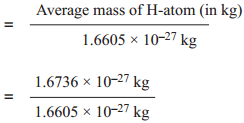Find free online Chemistry Topics covering a broad range of concepts from research institutes around the world.

## Atomic Mass | Definition, Units & Facts

How much does an individual atom weigh? As atoms are too small with diameter of 10-10 m and weigh approximately 10-27 kg, it is not possible to measure their mass directly. Hence it is proposed to have relative scale based on a standard atom.

The C-12 atom is considered as standard by the IUPAC (International Union of Pure and Applied Chemistry), and its mass is fixed as 12 amu (or) u. The amu (or) unified atomic mass unit is defined as one twelfth of the mass of a Carbon-12 atom in its ground state.

i.e. 1 amu (or) 1u ≈ 1.6605 × 10-27 kg.

In this scale, the relative atomic mass is defined as the ratio of the average atomic mass to the unified atomic mass unit.

Relative atomic mass (Ar)For example,

Relative atomic mass of hydrogen (Ar)H= 1.0078 ≈ 1.008 u.

Since most of the elements consist of isotopes that differ in mass, we use average atomic mass. Average atomic mass is defined as the average of the atomic masses of all atoms in their naturally occurring isotopes. For example, chlorine consists of two naturally occurring isotopes 17Cl35 and 17Cl37 in the ratio 77:23, the average relative atomic mass of chlorine is

= $$\frac{(35×77)+(37×23)}{100}$$
= 35.46 u